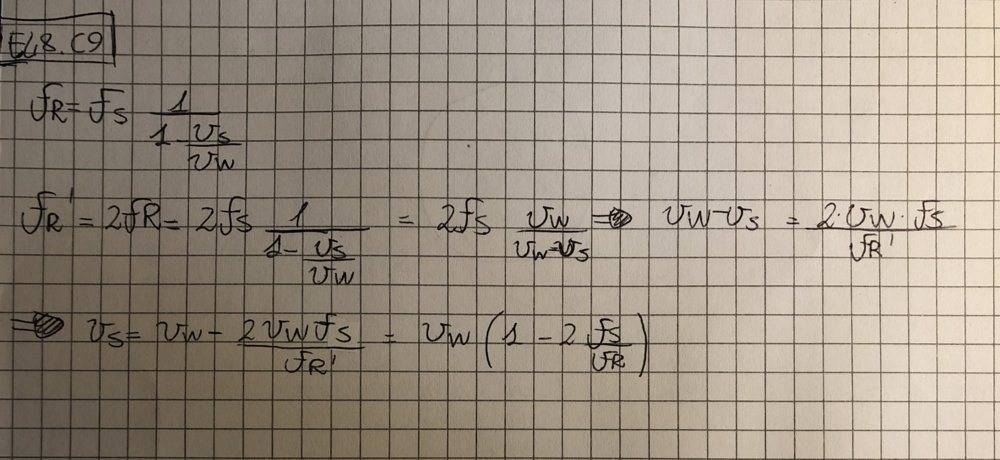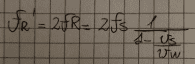# How to link frequency and speed? Doppler effect

Gold Member
Homework Statement:
At what speed should a plane move to make the roar of its turbines double in frequency for the observers who are in front of it in its path?
Does the result depend on the frequency considered?

RESULT: [vs = vw/2]
Relevant Equations:

CAPTION: "r = receiver", "s = source", "w = wave"
I had many attempts on trying to solve this one, but I got always stuck in the problem-solving part: how do I manage to find the source-speed from the Doppler formula, in an analytical way, and then reach to the result-formula?
Anyway, I'm pretty sure the only formula needed to solve this problem is the one i attached, which links the received-frequency to the source one, and the source/wave speed.

Homework Helper
Show your work to see where you get stuck...

Gold Member
Show your work to see where you get stuck...I hope to have attached the image in the right format and stuff, anyway, that's all I've written and where I exactly got stuck.
Starting from the basic Doppler formula, I just "flipped it around" a bit hoping to reach the resolutive one, but I lost my hopes when I found a totally different formula for vs, compared to the result one.

Mentor
I think that this is tripping you up:By doubling the source frequency on the right hand side you're not adjusting the received frequency via the speed of the source.

Instead, divide both sides of the original equation by fs so that you have a ratio of the two frequencies:
$$\frac{f_r}{f_s} = \frac{1}{1 - \frac{v_s}{v_w}}$$

Gold Member
I think that this is tripping you up:
View attachment 256505
By doubling the source frequency on the right hand side you're not adjusting the received frequency via the speed of the source.

Instead, divide both sides of the original equation by fs so that you have a ratio of the two frequencies:
$$\frac{f_r}{f_s} = \frac{1}{1 - \frac{v_s}{v_w}}$$

Yeah, you're definitely right, but I found out that the statement is bad formulated, since to solve the problem you need to assume that fR=2fS instead of what I've thought, and with this assumption I managed to solve it.